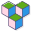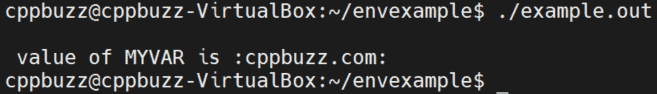CppBuzz# Example of Environment variables in Linux

1. export command is used to set environment variables.
2. ech \$variable is used to print value on command line.
3. getenv() library function is used to access the value from any C/C++ program.
4. We can view all environment variables using command 'env | more'.
5. 'unset' command is used to delete exported variales.
6. There should not be any space after = (i.e export MYVAR= cppbuzz.com is wrong, it should be export MYVAR=cppbuzz.com).

## Syntax to export environment variables

``````export MYVAR=cppbuzz.com
``````

## Print environment variables

``````echo \$MYVAR
``````

## Unset/Delete environment variables

``````unset MYVAR
``````

## Print all environment variables

``````env | more
``````## Accessing Environment variables in C++

getenv() is a library function which is used to read the env variables. Below example is in C++, but you can also call this function in C programming.

``````#include<iostream>
using namespace std;

int main()
{
cout<<"\n value of MYVAR is :"<<getenv("MYVAR")<<":"<<endl;
return 0;
}
``````